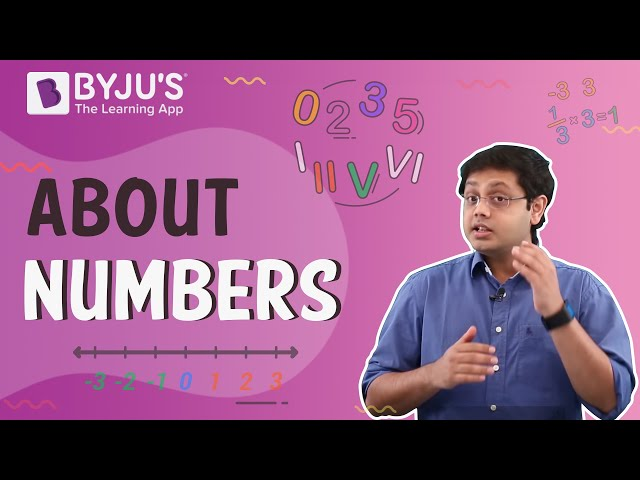Checkout JEE MAINS 2022 Question Paper Analysis : Checkout JEE MAINS 2022 Question Paper Analysis :

# Class 8 Maths Chapter 16 Playing with Numbers MCQs

Class 8 Maths Chapter 16 Playing with Numbers MCQs (Questions and Answers) are available online, with solved answers, for students. These objective questions for the 8th standard are designed by experts, according to the CBSE syllabus (2021-2022) and NCERT guidelines. The chapter-wise multiple-choice questions are provided at BYJU’S, for all students to learn the concepts thoroughly and score better marks in exams. Also, learn important questions for class 8 Maths here.

Practice more and test your skills on Class 8 Maths Chapter 16 Playing with Numbers MCQs with the given PDF here.

## MCQs on Class 8 Playing with Numbers with Answers

Multiple choice questions (MCQs) are given for Class 8 Playing with Numbers chapter.

1. The general form of abc is:

A. 100a + 10b + c

B. 100b + 10c + a

C. 100c + 10a + b

D. None of the above

2. The generalised form of 129 is:

A. 100+90+2

B. 100+20+9

C. 100+2+9

D. None of the above

3. The usual form of 100 × 7 + 10 × 1 + 8 is:

A. 108

B. 708

C. 718

D. 170

Explanation: 100 × 7 + 10 × 1 + 8

= 700 + 10 + 8

= 718

4. Which of the following numbers are not divisible by 5?

A. 20

B. 125

C. 122

D. 200

Explanation: To be completely divisible by 5, the number should have 0 or 5 at it’s one’s digit place.

5. Which of the following numbers are divisible by 10?

A. 99

B. 45

C. 110

D. 75

Explanation: If any number has 0 at its one’s digit place, then it is divisible by 10.

Hence, 110/10 = 11

6. Which of the following are divisible by 2?

A. 98

B. 99

C. 101

D. 121

Explanation: If one’s digit of any number is even number, then the number is completely divisible by 2.

Hence, 98/2 = 49

7. If a number is divisible 9, then it is divisible by:

A. 6

B. 7

C. 3

D. 11

Explanation: If 99 is divisible by 9 then it is also divisible by 3.

99/9 = 11 and 99/3 = 33

8. If the three digit number 24x is divisible by 9, the value of x is:

A. 3

B. 7

C. 1

D. None of the above

Explanation: If 24x is divisible by 9, then:

2+4+x = 6+x is also divisible by 9.

It is possible only when, 6+x = 9, 18, …

Since x is single digit, therefore, 6+3 = 9

x=3

9. The number 2146587 is divisible by:

A. 7

B. 3

C. 11

D. None of the above

Explanation: 2146587 = 2 + 1 + 4 + 6 + 5 + 8 + 7 = 33

Since, 33 is divisible by 3, hence 2146587 is divisible by 3.

10. The number 15287 is divisible by:

A. 3

B. 7

C. 9

D. None of the above

11. The general form of 1809 is:
A. 1 x 1000 + 8 x 100 + 9
B. 1800 + 9
C. 1000 + 809
D. None of the above

1809 = 1 x 1000 + 8 x 100 + 0 x 10 + 9

12. Which of the following numbers is divisible by 2?
A. 29
B. 19
C. 22
D. 47

Explanation:
22/2 = 11

13. Find the value of Q.
Q 1
+2 3

——-
4 4

——

A. 1
B. 2
C. 3
D. 4

2 1
+2 3
——–
4 4
_____

14. Suppose A is a digit. Find the value of A if 31A + 1A3 = 501.
A. 1
B. 2
C. 3
D. 4

Explanation:
318 + 183 = 501

15. Which value is divisible by 5?
A. 36
B. 90
C. 26
D. 81

Explanation: At the unit place of 90 we have 0. Hence, as per the divisibility rule for 5, 90 is divisible by 5.

16. Which of the following is divisible by 9?
A. 108
B. 107
C. 109
D. 105

Explanation: Sum of all digits of 108 = 1 + 0 + 8 = 9
9 is divisible by 9, thus, 108 is also divisible by 9.

17. Which of the following is divisible by 3?
A. 160
B. 170
C. 180
D. 190

Explanation: Sum of digits of 180 = 1 + 8 + 0 = 9
Thus, 9 is divisible by 3.

18. If the three-digit number 80x is divisible by 9, what is the value of x?
A. 1
B. 9
C. 7
D. 4

Explanation:

Given, 80x is divisible by 9.

Thus, sum of digits of 80x will also be divisible by 9.

8 + 0 + x is divisible by 9 only if, x = 1

8 + 0 + 1 = 9.

19. If the three-digit number 6 x 8 is divisible by 9, then what is the value of x?
A. 1
B. 2
C. 3
D. 4

Explanation: 6 x 8 → 6 + x + 8 = 14 + x
14 + x is divisible by 9
14 + 4 = 18 is divisible by 9

20. Which number is divisible by 10?
A. 89
B. 70
C. 75
D. 15